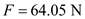# Three cylinders weighting 100 N each and of 80 mm diameter are placed in a channel of 180 mm width as shown in Fig. below Determine the pressure exerted by (i) the cylinder A on B at the point of contact (ii) the cylinder B on the base and (iii) the cylinder B on the wall.

Question-AnswerCategory: Engineering MechanicsThree cylinders weighting 100 N each and of 80 mm diameter are placed in a channel of 180 mm width as shown in Fig. below Determine the pressure exerted by (i) the cylinder A on B at the point of contact (ii) the cylinder B on the base and (iii) the cylinder B on the wall.

## Three cylinders weighting 100 N each and of 80 mm diameter are placed in a channel of 180 mm width as shown in Fig. below. Determine the pressure exerted by (i) the cylinder A on B at the point of contact (ii) the cylinder B on the base and (iii) the cylinder B on the wall.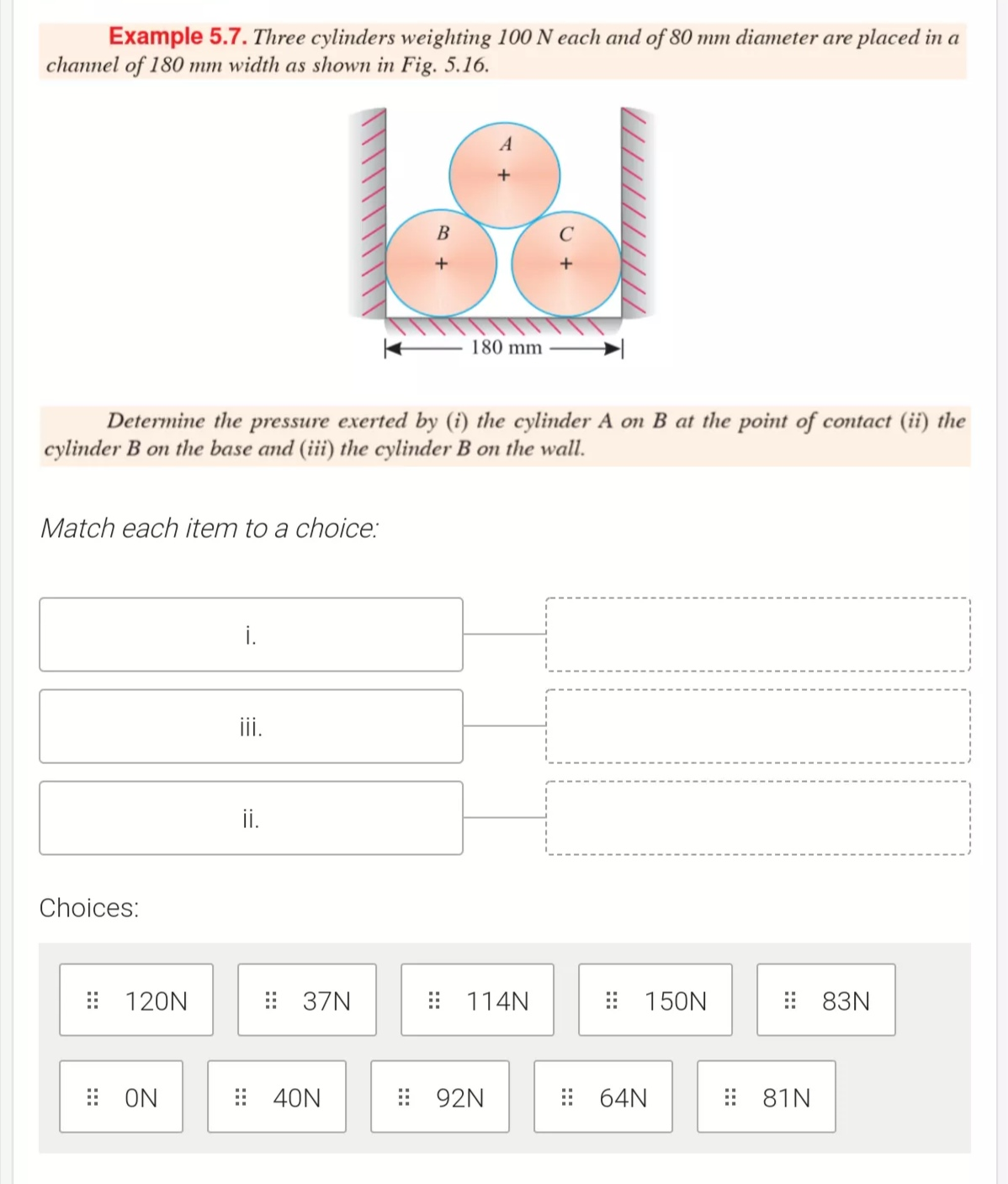Given,
weight of each cylinder=100N
Diameter of each cylinder=80mm

(i) Pressure exerted by cylinder A on B:

PQ=180-2r = 180-80=100
PS = 100/2= 50 mm
sin θ = PS/PR = 50/80
sinθ=0.625
θ=38.7

From lami’s theorem,

R1/sin(180-θ)=100/sin 2θ=R2/sin(180-θ)
R1sin/(180-38.7)=100/sin 2(38.7)
R1/0.625=100/0.9759
R1= 64N

Similarly, R2=64N because of same equation

(ii)Pressure exerted by cylinder  B on base

Rw: Reaction of B on vertical wall
Rb:Reaction of B on base

From free body diagram,
∑Fy = 0 = -R1cosθ + Rb=0
Rb=64 cos 38.7
Rb=50 N

(iii) Pressure exerted by B on  wall :

∑Fx = 0
=-R1sinθ + Rw=0
Rw=64 sin 38.7
Rw=40N

Consider pressure at the point of contact between cylinder A, B, and C is.Draw the free-body diagram of cylinder B showing the external forces.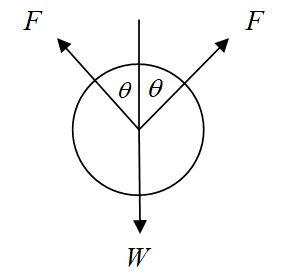Calculate the angle between the forces.Here, point A, B, and C represents cylinder centers.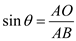Substitute the value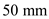for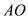andfor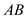.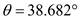Calculate the contact pressureacting on the cylinder B.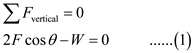Substitute,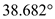for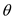and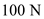for W in the equation (1).Hence, pressure exerted by cylindersand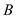at the point of contact is